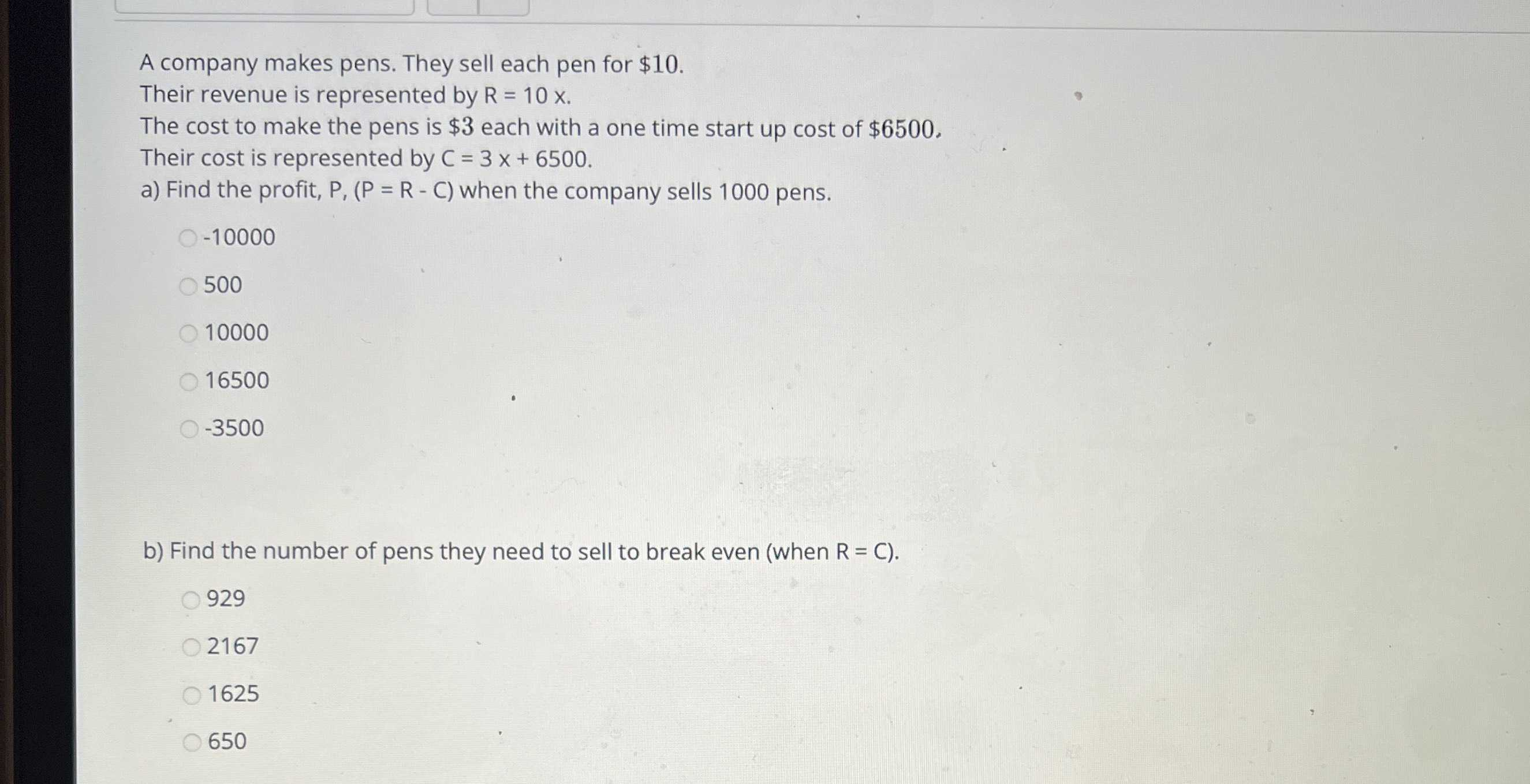### ¿Todavía tienes preguntas de matemáticas?

Pregunte a nuestros tutores expertos
Algebra
PreguntaA company makes pens. They sell each pen for $$\ 10$$ . Their revenue is represented by $$R = 10 x$$ . The cost to make the pens is $$\ 3$$ each with a one time start up cost of $$\ 6500$$ , Their cost is represented by $$C = 3 x + 6500$$ . a) Find the profit, $$P _ { 1 } ( P = R$$ - C) when the company sells $$1000$$ pens.

$$- 10000$$

$$500$$

$$10000$$

$$16500$$

$$- 3500$$

b) Find the number of pens they need to sell to break even (when $$R = C )$$ .

$$929$$

$$2167$$

$$1625$$

$$650$$